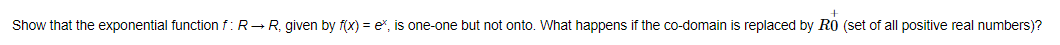# Show that the exponential function $f: R ightarrow R$, given by $f(x)=e^{x}$,

Question:Solution:

$f: R \rightarrow R$, given by $f(x)=\mathrm{e}^{x}$

Injectivity:
Let x and y be any two elements in the domain (R), such that f(x) = f(y)

$f(x)=f(y)$

$\Rightarrow e^{x}=e^{y}$

$\Rightarrow x=y$

So, is one-one.

Surjectivity:
We know that range of ex is (0, ∞) = R+
Co-domain = R
Both are not same.
So, f is not onto.

If the co-domain is replaced by R+, then the co-domain and range become the same and in that case, f is onto and hence, it is a bijection.